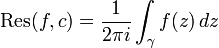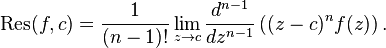# Residue and Singularity

## Homework Statement

z^-n(e^z-1)^-1 , z not equal to zero

locate the singularities and evaluate the residue.

## Homework Equations## The Attempt at a Solution

i don't have an idea about when z is not equal to zero because i think that only singularity point is z=0

hence if there is a special case, i dont have an idea, could you inform me. ? thanks.

In complex analysis, there are four kinds of singularity, to be described below. Suppose U is an open subset of the complex numbers C, and the point a is an element of U, and f is a complex differentiable function defined on some neighborhood around a, excluding a: U \ {a}.

* Isolated singularities: Suppose the function f is not defined at a, although it does have values defined on U \ {a}.
o The point a is a removable singularity of f if there exists a holomorphic function g defined on all of U such that f(z) = g(z) for all z in U \ {a}. The function g is a continuous replacement for the function f.
o The point a is a pole or non-essential singularity of f if there exists a holomorphic function g defined on U and a natural number n such that f(z) = g(z) / (z − a)n for all z in U \ {a}. The derivative at a non-essential singularity may or may not exist. If g(a) is nonzero, then we say that a is a pole of order n.
o The point a is an essential singularity of f if is neither a removable singularity nor a pole. The point a is an essential singularity if and only if the Laurent series has infinitely many powers of negative degree.

you should have LaTeX'd the original function.

assuming you mean $f(z)=\frac{1}{z^n} \frac{1}{e^{z-1}}$

we see that there is a pole of order n at z=0 as we need to multiply f(z) by (z-0)^n=z^n in order to remove this singularity. although if z isn't allowed to be equal to 0 then this isn't a problem.

however there will also be an essential singularity at z=1. this can be seen by expanding the exponential term. because this expansion goes on indefinitely i.e. there are an infinite number of terms, we need to multiply f(z) by (z-1) infinitely many times in order to remove the singularity i.e. the Laurent series has infinitely many powers of negative degree.

those formulas won't really be useful for calculating the residues. you'd be more likely to use them once you already know the residue to then go on and find the integral itself.

well the residue at z=a is defined as the coefficient of the $\frac{1}{z-a}$ term in the Laurent expansion.

thank you very much. i will try.

:)

i am going to inform u.

thanx again again again.

original function is z $$\overline{} -n$$ (e $$\overline{} -z$$ -1 ) $$\overline{} -1$$

original function is z $$\overline{} -n$$ (e $$\overline{} -z$$ -1 ) $$\overline{} -1$$

So, at what points does exp(-z) become equal to 1 (apart from z = 0)?

The residue at zero is difficult to compute directly via standard formulas. In fact, the series expansion of 1/(exp(-z) - 1) defines the so-called Bernouilli numbers for which you cannot write down a closed formula . But this exercise seeks a different expression.

If you first solve the first part of the problem and compute all the residues except at z = 0, you can consider the contour integral along a big circle of radius R and consider te limit of R to infinity.

Then you look at the limit of R to infinity of that contour integral. You can compute that easily. Then you know that this is also given as 2 pi i times the sum of all the residues inside the contour. You will see that you can compute the sum of all the residues that you've computed in terms of a special function. The residue at zero is the missing residue that you would have to add to that sum to get the limit of the contour integral.

You can also understand this method in terms of the so-called "residue at infinity". Suppose you have a contour integral along a contour that encoses the origin. Then, if you use the substitution z = 1/w in that contour integral you get a contour integral in the w-plane plane. However, the poles that were inside the contour in the z-plane are now outside the contour in the w-plane and vice versa. The sum of the residues for both functions must be the same. This gives the relation:

Sum of residues of f(z) = sum of residues of 1/w^2 f(1/w) (1)

On the left hand side we're summing over residues that are inside the contour in the z-plane, on the r..h.s. we take the residues that are inside the contour in the w-plane which correspond to the poles in the z-plane that are outside the contour.

Now you can formally compute the residue at a pole of 1/w^2 f(1/w) by considering a small contour around a pole. If we apply the transformation z to 1/z we find that it is given by minus the residue of f(z) at thae pole. That minus sign comes from
d(1/z) = -1/z^2 dz while the counterclockwise contour stays counterclockwise. If we encircle the origin, the orientation of the contour also changes, that's why we did not get the minus sign in formula (1).

So, Eq(1) seems to tell you that the sum of all the residues inside and outside the contour is equal to zero. But this can't be right, because then the limit of a contour integral along a circle of radius R would alwayus have to be zero. The mistake here is that on the right hand side of (1) there can be a residue at w = 0 and that doesn't correspond to any residue at some finite z. Of course w = 0 corresponds to z is infinity so a residue at w = 0 should be a residue at infinity in some sense. We know that at nonzero w you get minus the residue of f(z) at the corresponding z.

So, that's where the definition of the residue at infinity as the residue at w = 0 of -1/w^2 f(1/w) comes from. This then makes the sum of all the residues zero, if we also include the residue at infinity. Or, put differently, the residue at infinity is minus the sum of all the residues excluding the residue at infinity. And that you can compute by considering the limit of R to infinity of the contour integral over a circle of radius R. That limit can be seen as minus the residue at infinity.